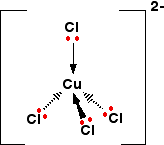# What is the cause of the color change observed when HCl is added to the complex ion [Cu(NH_3)_4]^(2+)?

Jul 18, 2017

I have explained a similar ligand displacement reaction to this in the answer below:

#### Explanation:

https://socratic.org/questions/hydrochloric-acid-copper-ll#415291

In this case the complex we have is tetraammine copper(II):If excess chloride ions are added the ammonia ligands are displaced and the green, tetrachloro copper(II) complex forms:

$\textsf{\textcolor{b l u e}{{\left[C u {\left(N {H}_{3}\right)}_{4} {\left({H}_{2} O\right)}_{2}\right]}^{2 +}} + 4 C {l}^{-} \rightarrow \textcolor{g r e e n}{{\left[C u C {l}_{4}\right]}^{2 -}} + 4 N {H}_{3} + 2 {H}_{2} O}$The solution looks like this: